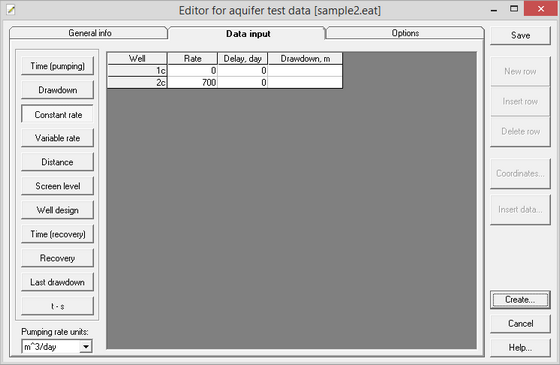﻿ ANSDIMAT+ > Data input (Editor) > Table data input > Constant rate

# Constant rate

Navigation:  ANSDIMAT+ > Data input (Editor) > Table data input >

# Constant rate

Input constant rate for all pumping wells. If pumping starts at different time in different wells, enter the delay of pumping for each well calculated from the start of pumping in the first well. The table is used for pumping test with  a constant rate. Rate dimension is selected from the combo box "Pumping rate units". During processing, ANSDIMAT automatically converts the rate to cubic meters per day.

This dialog box is also used for the constant drawdown test analysis.Dialog box for aquifer test data input: constant rate, constant drawdown.

Explanatory table

 "Well" column Names of pumping wells "Rate" column Constant pumping rate of each well. Pumping rate unit is chosen by the user. When user changes units, the input rate is automatically recalculated. "Delay" column Time delay (in days) between the start of pumping in the first well tested and the start of pumping in the current well. Relates to the multi-well test with asynchronous start of pumping wells "Drawdown" column Constant drawdown in pumping well. Applies to interpretation of a constant drawdown test "Rate dimension" combo box Select pumping rate unit: m3/day, m3/hour, m3/min, m3/sec, l/day, l/hour, l/min, l/sec. Changing units results in automatic recalculation of input pumping rate. ANSDIMAT converts the rate to cubic meters per day